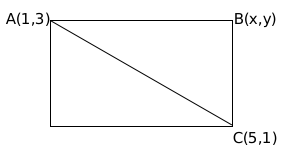Question 14

# ABCD is a rectangle in the clockwise direction. The coordinates of A are (1,3) and the coordinates of C are (5,1), the coordinates of vertices B and D satisfy the line $$y=2x+c$$, then what will be the coordinates of the mid-point of BC.

SolutionMidpoint of AC passes through the line y = 2x + c

Midpoint of AC = (3,2)

2 = 6 + c

c = -4

Line passing through B is y = 2x - 4

(slope of AB)(slope of BC) = -1

$$\left(\ \frac{\ y-1}{x-5}\right)\left(\ \frac{\ y-3}{x-1}\right)=-1$$

Substituting y = 2x - 4 and solving, we get

B(2,0) or B(4,4)

B cannot lie on X-axis. Therefore, co-ordinates of B = (4,4)

Midpoint of BC = $$\left(\frac{9}{2},\frac{5}{2}\right)$$